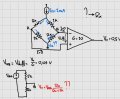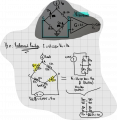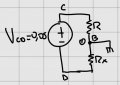# Wheatstone bridge excercise with Differential Amplifier

#### kalemaxon89

Joined Oct 12, 2022
217
In this exercise in the picture I have to find Rx.I have two questions:
1) Is it correct in the schematic that the blue current Ib (at the top) splits in the bridge and "joins" again at the bottom? (where GND is and I made the two question marks). Because, assuming the ideal amplifier, no current is flowing in its input terminals and therefore Ib must "flow out" somewhere, i.e., where there is ground.
2) I am stuck with carrying out the exercise (at the red formula) ... how do I go on? I'm having trouble figuring out if the bridge is balanced and continuing

#### WBahn

Joined Mar 31, 2012
29,508
Q1) Yes, the current splits at the top and rejoins at bottom, given the assumption that no current flows into the amplifier inputs.

Q2) If the bridge were balanced, what would be the differential voltage across the amplifier inputs? Is this consistent with the given output of the amplifier (i.e., 0.5 V)?

What is the total resistance down the left side of the bridge?

What is the total resistance down the right side of the bridge?

Given the answers to these two questions, what can you say about the current going down each side of the bridge, regardless of the value of Rx?

See if you can take it from there.

#### kalemaxon89

Joined Oct 12, 2022
217
Q1) Yes, the current splits at the top and rejoins at bottom, given the assumption that no current flows into the amplifier inputs.

Q2) If the bridge were balanced, what would be the differential voltage across the amplifier inputs? Is this consistent with the given output of the amplifier (i.e., 0.5 V)?

What is the total resistance down the left side of the bridge?

What is the total resistance down the right side of the bridge?

Given the answers to these two questions, what can you say about the current going down each side of the bridge, regardless of the value of Rx?

See if you can take it from there.Last edited:

#### kalemaxon89

Joined Oct 12, 2022
217
Q1) Yes, the current splits at the top and rejoins at bottom, given the assumption that no current flows into the amplifier inputs.

Q2) If the bridge were balanced, what would be the differential voltage across the amplifier inputs? Is this consistent with the given output of the amplifier (i.e., 0.5 V)?

What is the total resistance down the left side of the bridge?

What is the total resistance down the right side of the bridge?

Given the answers to these two questions, what can you say about the current going down each side of the bridge, regardless of the value of Rx?

See if you can take it from there.
Maybe it's easier than expected and you simplify everything this way: